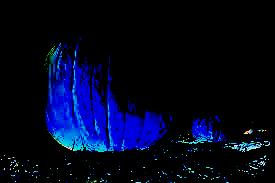# Wand function() function in Python

The Wand function() function in Python has a list of function_types to choose from and edit your images. You can apply these to personalize your images. In technical terms, the pixel channels can be manipulated by applying a multi-argument function to pixel channels. There are 5 function_types to apply to your image.
The Wand is a ctypes-based simple ImageMagick binding for Python, supporting 2.6+ and 3.3+. All functionalities of MagickWand API are implemented in Wand. You can use this in your Python program using the import statement. But you need to install it first in your system .

## How to install

To install Wand in your system, run command prompt and type these commands one by one.

```\$ apt-get install libmagickwand-dev
\$ pip install Wand```

You can refer here for the installation process in your system(Windows, Mac, Linux).

## Function_types

Here is a list of function_types you can choose from and personalize your images according to your preference.

• ‘undefined’
• ‘arcsin’
• ‘arctan’
• ‘polynomial’
• ‘sinusoid’

## Implementation

• First, you need to import the wand function in your Python program using,
`from wand.image import Image`
• You need to define some variables like frequency, phaseShift, amplitude, and bias.
• Then, using with you can use Image() function that was imported at the beginning of the program and you need to pass the filename inside the function.
• Here, i.function() refers to the wand function() function inside which we need to pass function_type like polynomial in program-1 and also the variables which we have defined at the start of this program.
• You can play around with these variables and can have different results for your image.
• Lastly, you need to save your resultant image using i.save() function inside which we need to pass the filename.

The image that I am using here is,Program-1

```from wand.image import Image

frequency = 5
phaseShift = -80
amplitude = 0.5
bias = 0.9

#Using polynomial function_type
with Image(filename = "pumpkin.jpeg") as i:
i.function('polynomial', [frequency, phaseShift, amplitude, bias])
i.save(filename = "pumpkin-4.jpeg")
```

Output-1Program-2

```from wand.image import

Image frequency = 5
phaseShift = -80
amplitude = 0.5
bias = 0.9

#Using sinusoid function_type
with Image(filename = "pumpkin.jpeg") as i:
i.function('sinusoid', [frequency, phaseShift, amplitude, bias])
i.save(filename = "pumpkin-5.jpeg")```

Output-2